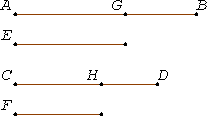# Proposition 25

If four magnitudes are proportional, then the sum of the greatest and the least is greater than the sum of the remaining two.

Let the four magnitudes AB, CD, E, and F be proportional so that AB is to CD as E is to F, and let AB be the greatest of them and F the least.

I say that the sum of AB and F is greater than the sum of CD and E.Make AG equal to E, and CH equal to F.

Since AB is to CD as E is to F, and E equals AG, and F equals CH, therefore AB is to CD as AG is to CH.

V.19

And since the whole AB is to the whole CD as the part AG subtracted is to the part CH subtracted, therefore the remainder GB is also to the remainder HD as the whole AB is to the whole CD.

V.Def.5

But AB is greater than CD, therefore GB is also greater than HD.

And, since AG equals E, and CH equals F, therefore the sum of AG and F equals the sum of CH and E.

And if, GB and HD being unequal, and GB greater, the sum of AG and F is added to GB, and the sum of CH and E is added to HD, it follows that the sum of AB and F is greater than the sum of CD and E.

Therefore, if four magnitudes are proportional, then the sum of the greatest and the least is greater than the sum of the remaining two.

Q.E.D.

## Guide

This proposition says that if w : x = y : z and w is the greatest of the four magnitudes while z is the least, then w + z > x + y. All four magnitudes must be of the same kind.

The argument goes as follows. Since w : x = y : z, therefore (w – y) : (x – z) = w : x, by V.19. But since w > x, therefore w – y > x – z. Thus w + z > x + y.

This proposition is not used in the rest of the Elements but is an end in itself.

#### Arithmetic and geometric means

A special case of this proposition occurs when the middle terms are the same: x : y = y : z. In that case y is the mean proportional, equivalent to the geometric mean for real numbers and described as the square root of the product xz. The conclusion of the proposition, after dividing by 2, says (x + z)/2 > y. The arithmetic mean, or average, of two magnitudes is half their sum. Thus, this proposition gives as a corollary
The arithmetic mean of two magnitudes is less than their geometric mean.

This proposition relies on treating V.Def.4 as an axiom of comparability. Infinitesimal counterexample: when y is infinitesimal with respect to x, consider the proportion (x + 5y) : (x + 2y) = (x + 4y) : x.